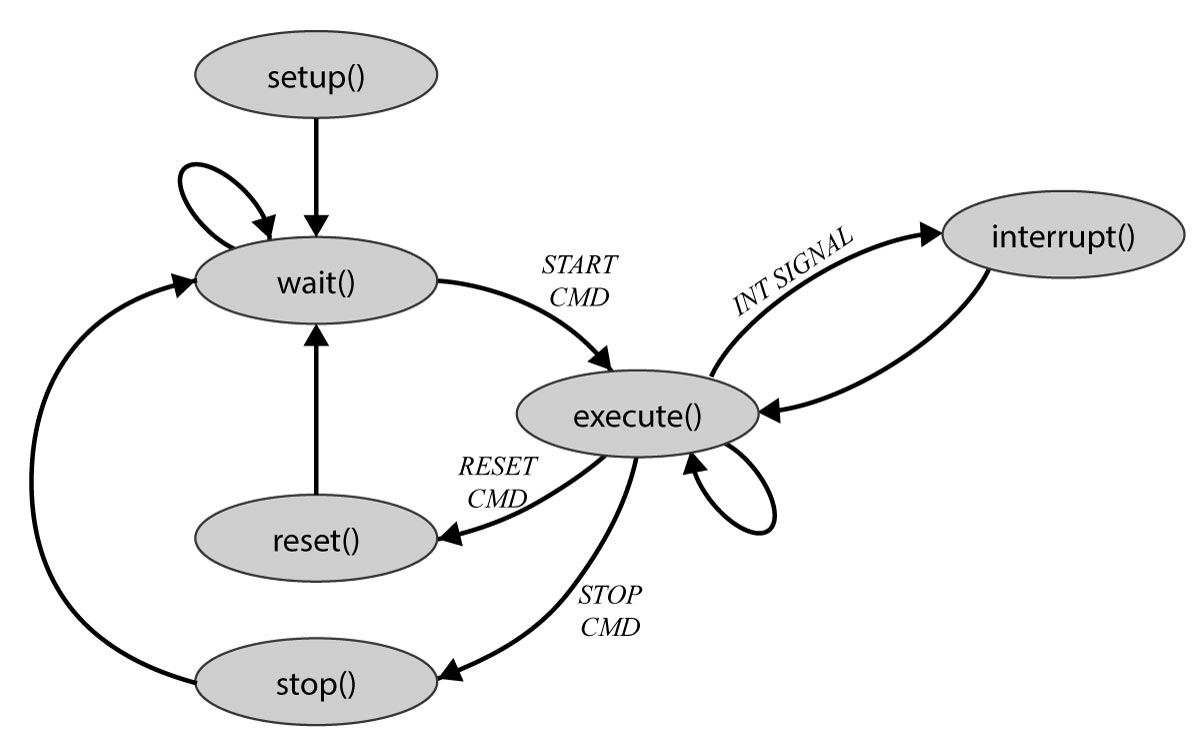# Robot Firmware Architecture

The firmware of the robot was implemented using PlatformIO, based on the ESP32 wrapper of the Arduino Framework. PlatformIO environment is available on almost all major IDEs, and once installed, it will do all the dependency management for you.

Developers who want to model the Swarm Behavioral Algorithms doesn’t need to worry about the implementations of the hardware. They can use the top level implementation, which be found in firmware\algorithms\algorithm_template.cpp of the firmware repository.

### Template for the Algorithm Implementation

``````#include "algorithm.h"
#include "config/global_variables.h"
#include "mqtt/mqtt.h"

#ifdef ALGO_TEMPLATE // replace this with the name  of your algorithm
// -----------------------------------------------------------------------------

int robotState = ROBOT_BEGIN;

// Define your global variables here -----

// ---------------------------------------

void algorithm_setup(){
Serial.println("algorithm: setup");
// Define what need to be setup in here...

}

void algorithm_loop(){
// Robot state machine is defined in here

if(robotState==ROBOT_RUN){
algorithm_execute();
delay(50);

}else if(robotState==ROBOT_BEGIN){
algorithm_setup();
robotState = ROBOT_WAIT;

}else{
// wait
delay(100);
}
}

void algorithm_interrupt(robot_interrupt_t interrupt, char* msg){
// Define Interrupt Handlers in here

if(interrupt == INT_COMM_IN){
// Communication Interrupt
}
}

void algorithm_execute(){
// Deine the algorithm in here...
}

// instruct to start the pattern
void algorithm_start(){
robotState = ROBOT_RUN;
Serial.println("algorithm: start");
// Define the tasks to be done at the start of the algorithm in here...
}

void algorithm_reset(){
// Define the tasks to be done at reset in here...

robotState = ROBOT_BEGIN;
Serial.println("algorithm: reset");
}

void algorithm_stop(){
// Define the tasks to be done to stop an algorithm in here...

robotState = ROBOT_WAIT;
Serial.println("algorithm: wait");
}

#endif
``````

Please make sure to change the following line of the Template, once you created a new algorithm file on the folder.

``````// replace this with the name of your algorithm
#ifdef ALGO_TEMPLATE
``````

After, please visit the /firmware/features.h file and, and add the name you used as a new #define statement and uncomment it, while commenting all other ALGO_* lines.

``````// Can use 'ONLY ONE' of the following algorithms
// #define ALGO_COLOR_RIPPLE
// #define ALGO_MOVE_ROBOT
#define ALGO_DISCOVER_COLOR
// #define ALGO_COMMUNICATION
``````

There are a few sample implementations are available from below links:

The following image, the Finite State Machine describes when and how each function in the algorithm template will be executed.``````// Algorithm setup
void algorithm_setup();

// Algorithm loop
void algorithm_loop();

// Algorithm should be implemented on here
void algorithm_execute();

// Invoke a software interrupt for the robot, when a message arrived
void algorithm_interrupt(robot_interrupt_t interrupt, char* msg);

// instruct to start the experiment
void algorithm_start();

// reset the experiment variables and the state
void algorithm_reset();

// stop the execution of the experiment
void algorithm_stop();
``````